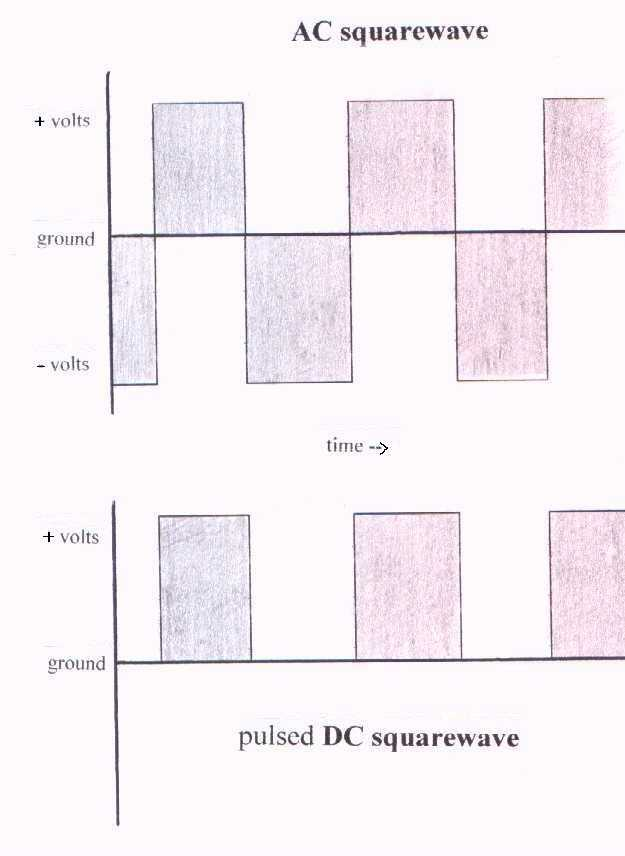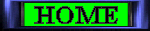Electronics Terminology Voltage is the electrical force that creates electrical current. The unit of measure is volts. Current is the directional movement of electrons. The unit of measure is amperes (A).  The human body can only comfortably tolerate thousandths (1/1000) of an amp (ampere). The abbreviation for a thousandth is 'milliamp (mA)'. One milliamp (1mA) is .001 amps. The abbreviation for a millionth is 'microamp (uA)'. One micro-amp (1uA) is .000001 amps. If a blood electrification unit puts out 130uA then it is outputting .00013 amps. Direct current (DC) flows in only one direction. Alternating current (AC) continually changes directions back and forth at a rate set by the frequency. Resistance is that which 'resists' the flow of electrons. The unit of measure is ohms. People that are mineral deficient have more resistance to current flow and therefore have to apply more voltage to get the same amount of current as a normal person. The output control on many units is just a variable resistor that causes a greater resistance to current flow as the knob is turned counterclockwise. Frequency is the rate of waveform repetition. This rate is expressed as cycles-per-second. If a waveform goes from 0 volts to 30 volts and back to zero 30,000 times a second then '30,000 hertz (Hz)' is the frequency. 30,000 can be abbreviated as '30Khz' since 'K' represents '1000'. ('M' is '1,000,000'). Waveform is the 'form' of the voltage waveshape being output by a unit. A square wave is the normal waveshape for most electromedicine devices. Its voltage level changes occur instantly and therefore looks square-ish when viewed by an oscilloscope (a visual device that shows the real-time plot of the output with voltage being the vertical scale and time being the horizontal scale). Other considerations: The voltage output of units can be 'pulsed DC' or 'AC'. Pulsed DC changes voltage from zero to a positive voltage and back to zero according to the frequency rate. AC changes voltage from positive to negative and back to positive according to the frequency rate. An AC output can have its maximum voltage output expressed as 'peak voltage' (the maximum voltage from ground) or 'peak-to-peak voltage' (the voltage difference from the most positive to the most negative). So an AC unit can be said to output 30 volts peak, or 60 volts peak to peak. Clark zappers output pulsed DC that changes from zero to positive 5 volts so they can be said to output 5 volts peak.Waveforms